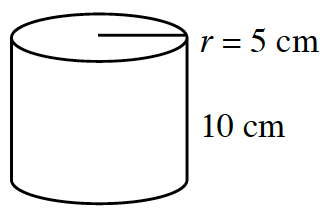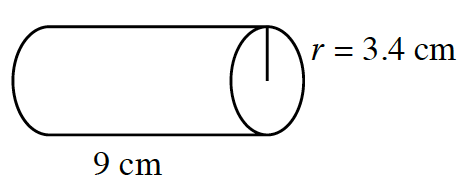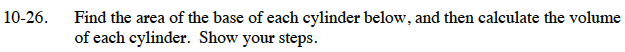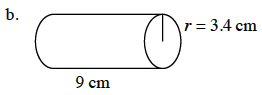Home > ACC7 > Chapter cc310 > Lesson cc310.1.2 > Problem10-26

10-26.
1. Find the area of the base of each cylinder below, and then calculate the volume of each cylinder. Show your steps. Homework Help ✎

1.2.The base is a circle, so find the area of the circle using the following equation:
Area = π(r2)

Area = π(5²)

Area = 25π cm² or approximately 78.54 cm².
Now use the equation for the volume of a cylinder:
Volume = (area of base) (height)

Volume = (78.54)(10)

785.4 cm³See part (a).